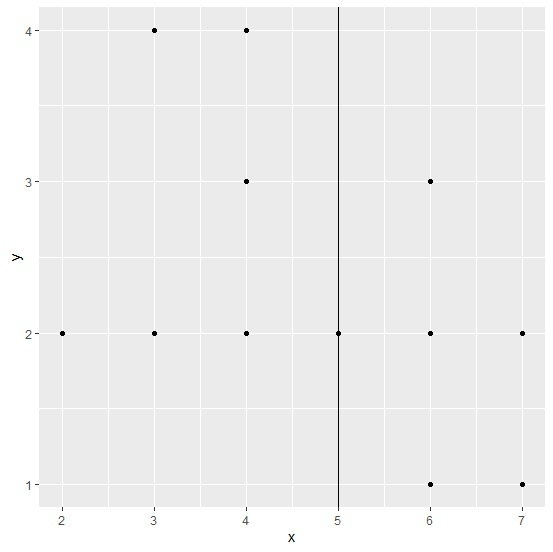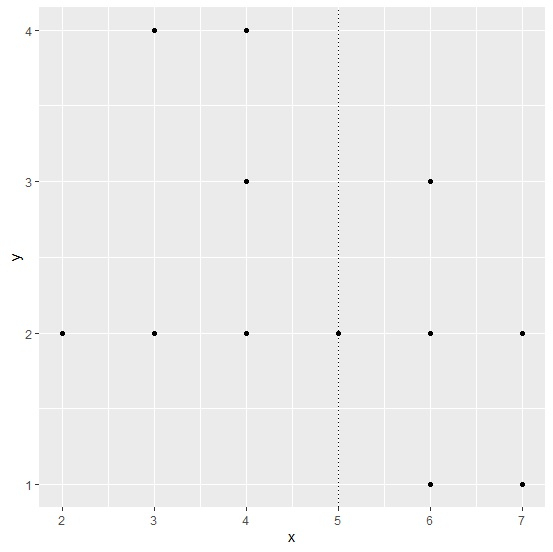# How to create a dotted vertical line using ggplot2 in R?

To create a vertical line using ggplot2, we can use geom_vline function of ggplot2 package and if we want to have a dotted vertical line then linetype will be set to 3 inside the same function. To draw the line, we will have to provide xintercept because the line will start on X-axis.

Check out the below example to understand how it works.

## Example

Following snippet creates a sample data frame −

x<-rpois(20,5)
y<-rpois(20,2)
df<-data.frame(x,y)
df

The following dataframe is created −

   x  y
1  5  2
2  3  4
3  6  2
4  3  4
5  4  2
6  5  2
7  7  1
8  4  2
9  3  2
10 4  4
11 6  2
12 2  2
13 7  1
14 7  1
15 6  1
16 7  1
17 7  2
18 7  1
19 6  3
20 4  3

To load ggplot2 package and create point chart between x and y with vertical line at X=5, add the following code to the above snippet −

library(ggplot2)
ggplot(df,aes(x,y))+geom_point()+geom_vline(xintercept=5)

## Output

If you execute all the above given snippets as a single program, it generates the following output −Now, to create point chart between x and y with dotted vertical line at X=5, add the following code to the above snippet −

ggplot(df,aes(x,y))+geom_point()+geom_vline(xintercept=5,linetype=3)


## Output

If you execute all the above given snippets as a single program, it generates the following output −